• matlab实现图像对比度增强算法的源代码，很实用。
• 采用有效的对比度增强技术被认为是改善模糊图像整体视觉表现的必要步骤。 这项工作利用一种简单快速的技术，通过将该技术直接应用于整个图像并根据其在空间域中的大小对其进行归一化，来提高不同类型图像的亮度和...
• matlab开发-用于增强图像对比度的不清晰度屏蔽滤波器的自适应比例调整设计。图像对比度增强非锐化掩模滤波器的自适应尺度调整设计
• 通过直方图均衡技术（RMSHE、ClaHE 等）增强图像对比度
• matlab开发-彩色图像对比度增强。这个程序演示了色彩空间中对比度增强的概念。
• matlab开发-图像对比度增强，使用最大亮度强度的灰度质量。最大强度覆盖直方图均衡增强图像对比度
• matlab开发-BrightnessPreservationBasedOnDynamicStretching的图像对比度增强。基于动态拉伸的图像对比度增强亮度保持
• 对彩色图像进行增强、主要增强两方面，一方面是图像的亮度，另一方面就是图像对比度。 参考链接：https://www.jianshu.com/p/5a8d12d6c649 基于修改直方图的方法： 将RGB格式的图像转为HSV或者HSI格式，对于亮度...
对彩色图像进行增强、主要增强两方面，一方面是图像的亮度，另一方面就是图像的对比度。
参考链接：https://www.jianshu.com/p/5a8d12d6c649
基于修改直方图的方法：

将RGB格式的图像转为HSV或者HSI格式，对于亮度值进行修改。主要是对V值直方图进行均衡化。

clear all;

close all;

RGB=imread('test.jpeg');

HSV=rgb2hsv(RGB);

H=HSV(:,:,1);

S=HSV(:,:,2);

V=HSV(:,:,3);

figure;

subplot(1,3,1),imhist(H);

subplot(1,3,2),imhist(S);

subplot(1,3,3),imhist(V);

V=histeq(V);

figure,imhist(V);

HSV(:,:,1)=H;

HSV(:,:,2)=S;

HSV(:,:,3)=V;

RGB_1=hsv2rgb(HSV);

figure;

subplot(1,2,1),imshow(RGB);

subplot(1,2,2),imshow(RGB_1);

作者：amazingu
链接：https://www.jianshu.com/p/5a8d12d6c649
来源：简书
著作权归作者所有。商业转载请联系作者获得授权，非商业转载请注明出处。

补充：
以下三个函数特别适用于对比度增强：imadjust、histeq 和 adapthisteq。

imadjust 将输入强度图像的值映射到新值，以对输入数据中强度最低和最高的 1%（默认值）数据进行饱和处理，从而提高图像的对比度。
histeq执行直方图均衡化。它变换强度图像中的值，以使输出图像的直方图近似匹配指定的直方图（默认情况下为均匀分布），从而增强图像的对比度。
adapthisteq 执行对比度受限的自适应直方图均衡化。与 histeq不同，它对小数据区域（图块）而不是整个图像执行运算。它会增强每个图块的对比度，使得每个输出区域的直方图近似匹配指定的直方图（默认情况下为均匀分布）。可以限制对比度增强，以避免放大图像中可能存在的噪声。


展开全文• matlab开发-图像对比度增强亮度保留最佳算法修正和加权总和。基于最优伽玛校正和加权和的图像对比度增强亮度保持
• LCC算法全名local color correction，用于增强图像对比度，英文全文在IPOL可以下载。 算法设计思路 计算输入图像的mask image 将输入与mask image进行计算，完成2幅图像的组合 Matlab实现 下面给出Matlab实现的...
目录ISP  Matlab 图像对比度增强——LCC算法学习算法设计思路Matlab实现测试
ISP  Matlab 图像对比度增强——LCC算法学习
LCC算法全名local color correction，用于增强图像的对比度，英文全文在IPOL可以下载。
算法设计思路

计算输入图像的mask image
将输入与mask image进行计算，完成2幅图像的组合

Matlab实现
下面给出Matlab实现的主体部分，比较简单。
%% 算法参数配置
Rad = 2;
sigma = 2;
filter_kernel1 = fspecial('gaussian',2*Rad+1 , sigma);
pix_width = 8;
pix_max = 2^pix_width;
pix_mid = 2^(pix_width-1);

%% 算法主体
mask_img = (img_in(:,:,1) + img_in(:,:,2) + img_in(:,:,3))./3;
mask_img = pix_max - mask_img;
gaus_out = imfilter(mask_img(:,:,:),filter_kernel1,'symmetric');
img_out = zeros(m, n, d);

test = (2.^((pix_mid-gaus_out(:,:,:))./pix_mid));

for ch =1:1:3
img_out(:,:,ch) = pix_max.*(img_in(:,:,ch)./pix_max).^(2.^((pix_mid-gaus_out(:,:,:))./pix_mid));
end


测试
采用莫里斯大教堂作为测试输入，分别选择了一副稍亮和一副偏暗的图像送入算法进行处理，在输入图像动态范围较宽时，测试算法自身的适应型能力。

测试用例1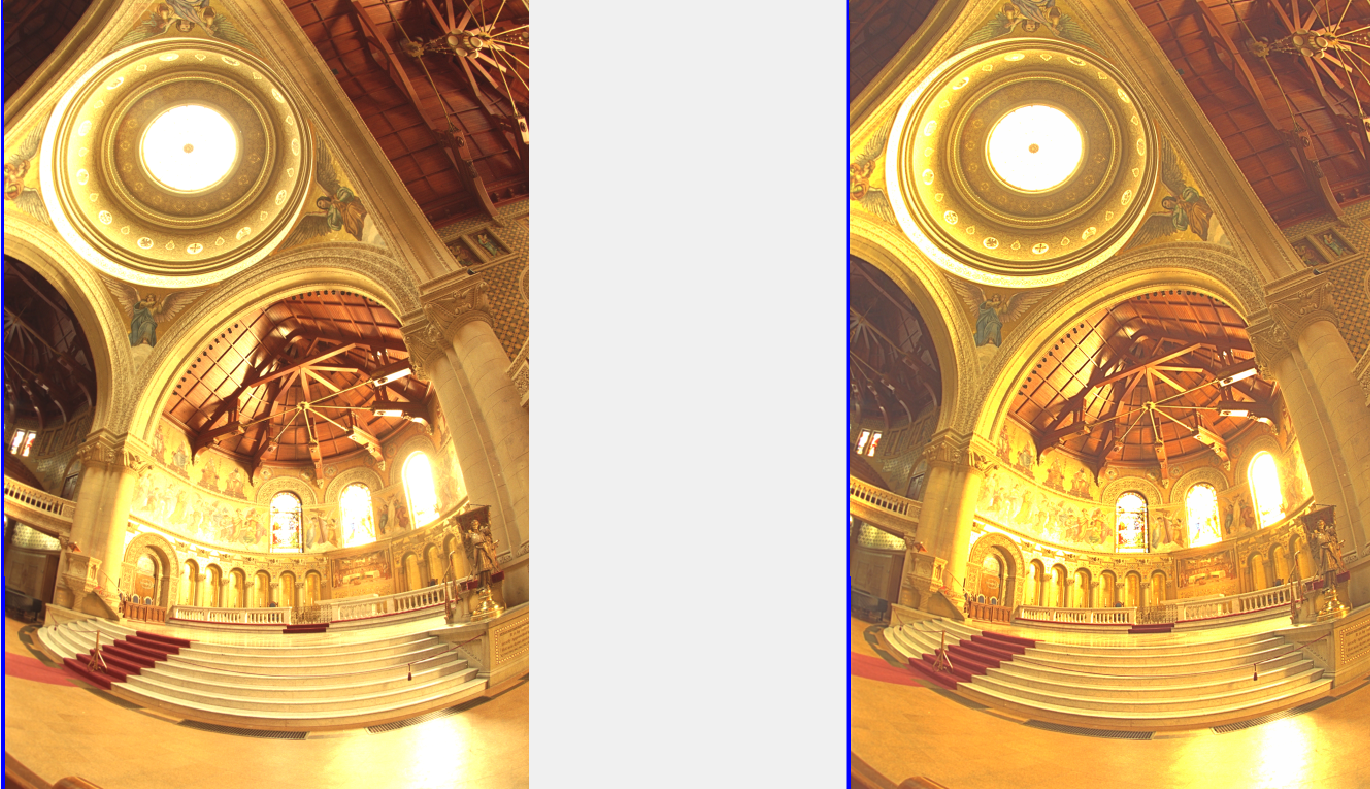测试用例2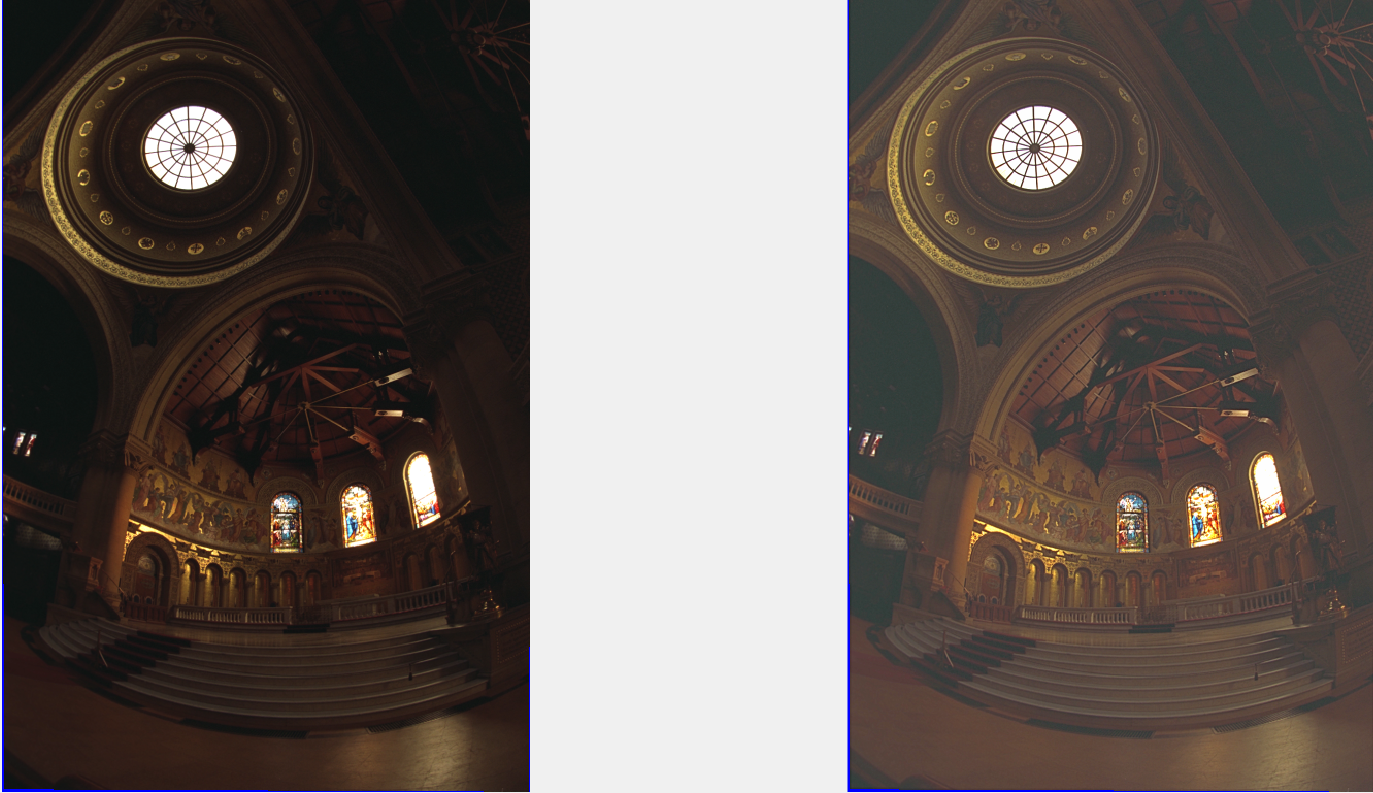针对2幅场景， 该算法均对图像有一定的改善，实际针对视频场景需要评估。


展开全文算法
• 图像增强的方法分类：1 从处理...邻域方法，即空域滤波），频域方法4 从处理目的分类：图像锐化，平滑去噪，灰度调整（对比度增强）二 图像增强的方法之对比度增强1 灰度变换法线性变换（已实现）对数变换（已实...
一 图像增强的方法分类：1 从处理对象分类：灰度图像，（伪）彩色图像2 从处理策略分类：全局处理，局部处理（ROI ROI，Region of Interest Interest）3 从处理方法分类：空间域（点域运算，即灰度变换；邻域方法，即空域滤波），频域方法4 从处理目的分类：图像锐化，平滑去噪，灰度调整（对比度增强）二 图像增强的方法之对比度增强1 灰度变换法线性变换（已实现）对数变换（已实现）指数变换（已实现）2 直方图调整法直方图均衡化（已实现）  Histogram Equalization.直方图匹配（未实现）Fr： https://blog.csdn.net/liuxiangxxl/article/details/78657244
展开全文• 此功能允许设置全局图像强度阈值，从而导致对比度增强（按颜色量化的价格）。 这是一种原始的图像判别方式元素基于它们的颜色 - 增加一些，减少其他，根据它们与阈值的关系。 该贡献还包括一个包含此功能的小型 GUI...
• 点运算又称为对比度增强对比度拉伸或灰度变换，是一种通过图像中的每一个像素值进行运算的图像处理方式。。它将输入图像映射为输出图像，输出图像每个像素点的灰度值仅有对应的输入像素点的灰度值决定，运算结果...
点运算又称为对比度增强、对比度拉伸或灰度变换，是一种通过图像中的每一个像素值进行运算的图像处理方式。。它将输入图像映射为输出图像，输出图像每个像素点的灰度值仅有对应的输入像素点的灰度值决定，运算结果不会改变图像内像素点之间的空间关系。其运算的数学关系式如下：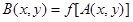其中，A（x，y）表示原图像，B（x，y）表示经过点运算处理后的图像，f表示点运算的关系函数。按照灰度变换的数学关系，点运算可以分为线性灰度变换、分段线性灰度变换和非线性灰度变换3种。接下来我们分别举例实现。1. 线性灰度变换假定原图像A（x，y）的灰度变换范围为[a，b]，处理后的图像B（x，y）的灰度变换范围为[c，d]，线性灰度变换运算的数学表达式为：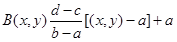在MATLAB图像处理工具箱中提供了一个灰度线性变换函数imadjust（）close all;clear all;clc;
%通过函数imadjust()对图像进行线性灰度变换
gamma=0.5;  %设定调整线性度取值
I=imread('F:/pao1.jpg');
R=I;  %将图像数据赋值给R
R(:,:,2)=0;  %将原图像变成单色图像，保留红色
R(:,:,3)=0;
R1=imadjust(R,[0.5 0.8],[0 1],gamma);  %利用函数imajust（）调整R的灰度，结果返回R1
G=I;
G(:,:,1)=0;  %将原图像变成单色图像，保留绿色
G(:,:,3)=0;
G1=imadjust(G,[0 0.3],[0 1],gamma);  %利用函数imajust（）调整G的灰度，结果返回G1
B=I;
B(:,:,1)=0;  %将原图像变成单色图像，保留蓝色
B(:,:,2)=0;
B1=imadjust(B,[0 0.3],[0 1],gamma);  %利用函数imajust（）调整B的灰度，结果返回B1
I1=R1+G1+B1;  %求变换后的RGB图像
figure,
subplot(131),imshow(I);
subplot(132),imshow(R);
subplot(133),imshow(R1);
figure,
subplot(131),imshow(I);
subplot(132),imshow(G);
subplot(133),imshow(G1);
figure,
subplot(131),imshow(I);
subplot(132),imshow(B);
subplot(133),imshow(B1);
figure,
subplot(121),imshow(I);
subplot(122),imshow(I1);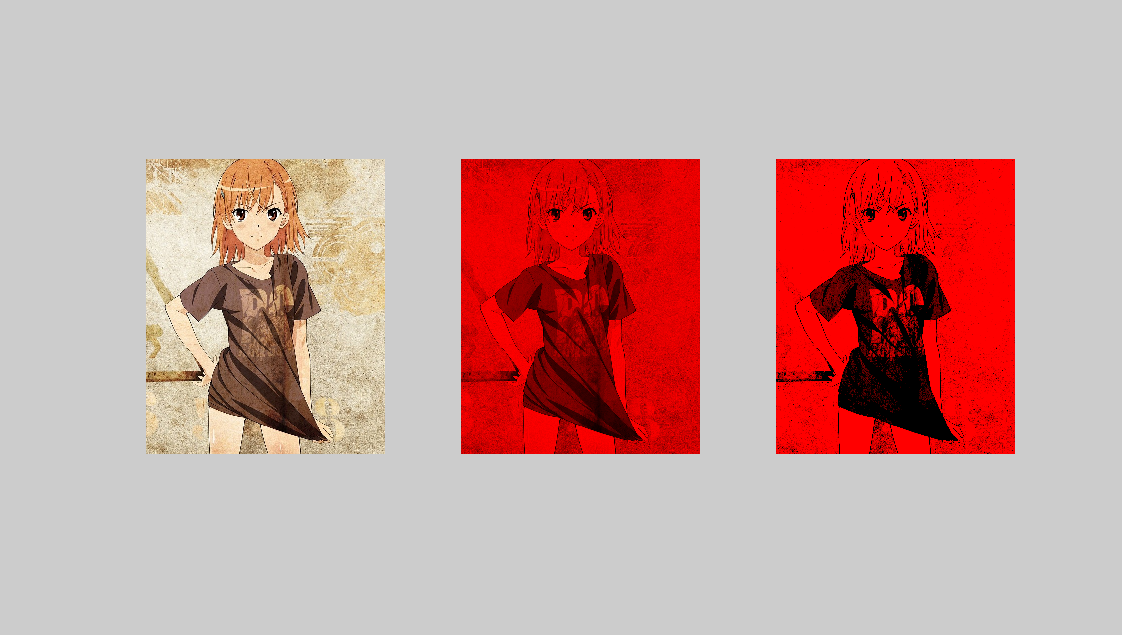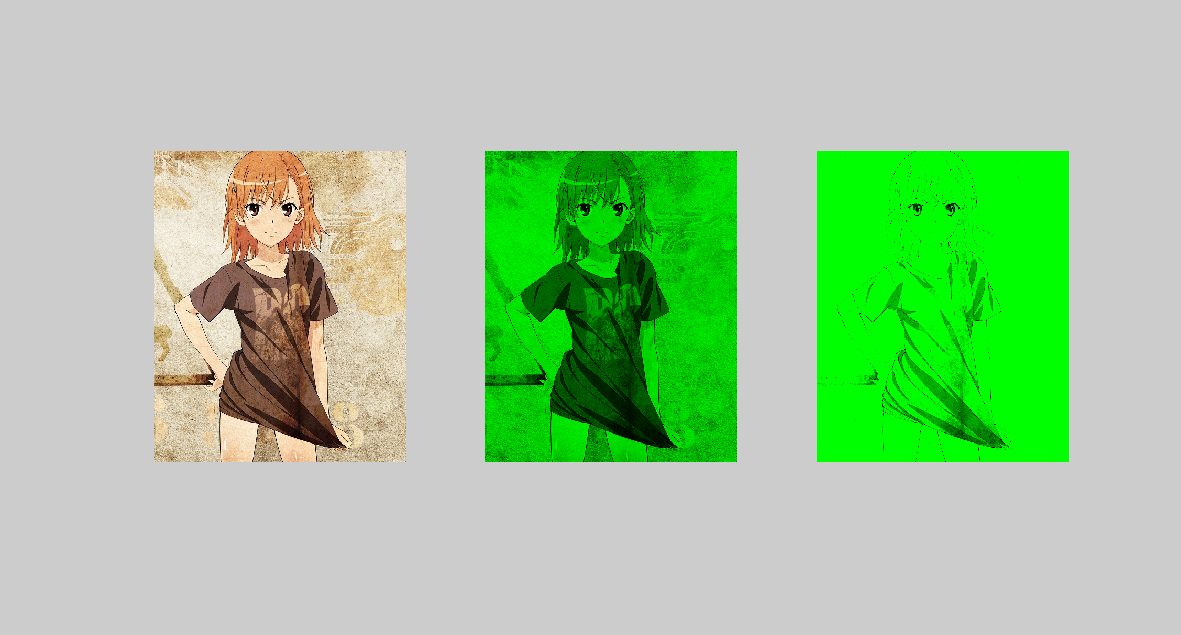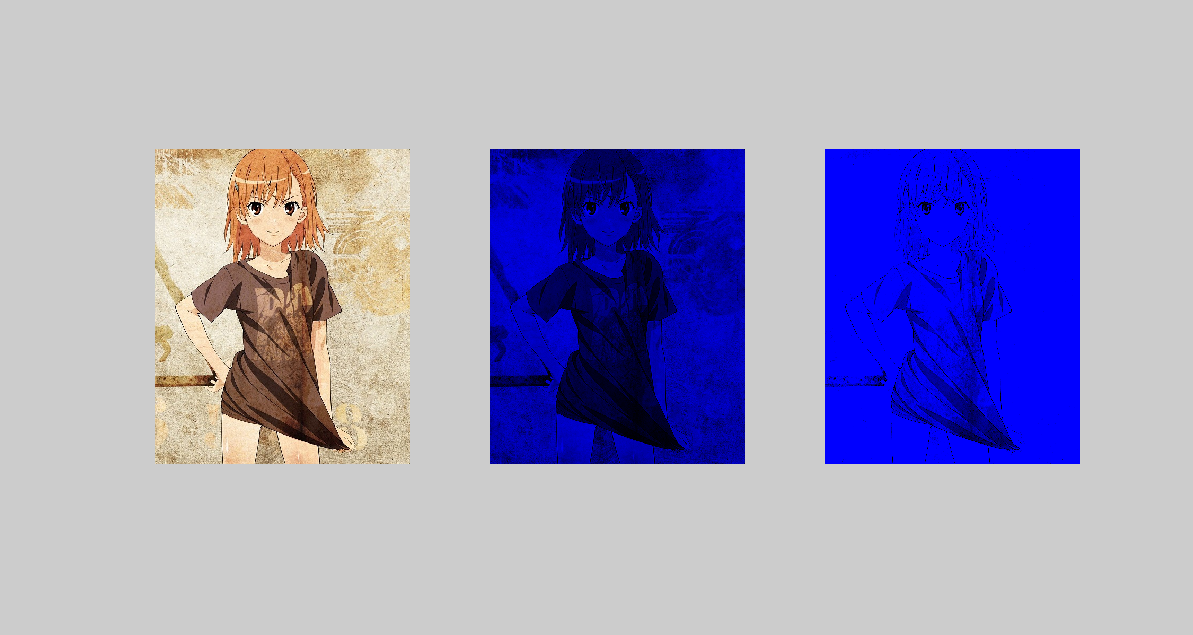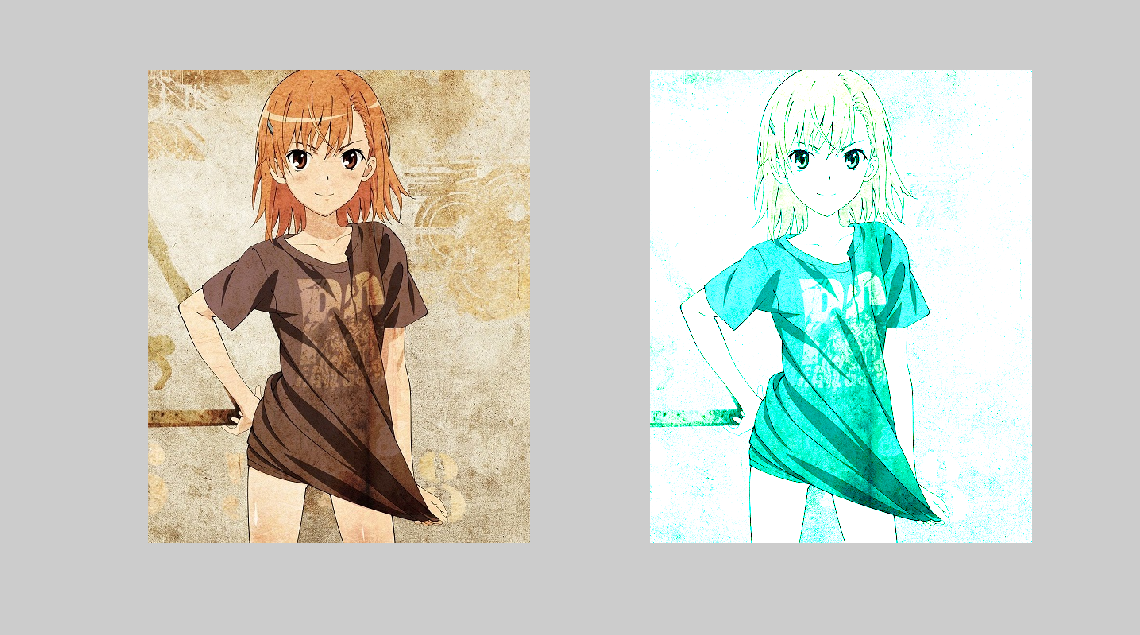2. 分段线性灰度变换为了突出图像中感兴趣的目标或者灰度区间，可采用分段线性法，将需要的图像细节灰度拉伸，对比度增强。3段线性变换法运算的数学表达式如下：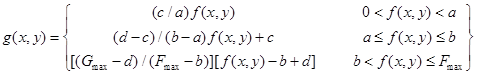close all;clear all;clc;
%分段线性灰度变换
R=imread('F:/pao1.jpg');
J=rgb2gray(R);  %将彩色图像数据R转换为灰度图像数据J
[M,N]=size(J);  %获得灰度图像J的行列数M，N
x=1;y=1;
for x=1:M
for y=1:N
if (J(x,y)<=35);  %对灰度图像J进行分段处理，处理后的结果返回给矩阵H
H(x,y)=J(x,y)*10;
elseif(J(x,y)>35&J(x,y)<=75);
H(x,y)=(10/7)*[J(x,y)-5]+50;
else(J(x,y)>75);
H(x,y)=(105/180)*[J(x,y)-75]+150;
end
end
end
figure,
subplot(121),imshow(J);
subplot(122),imshow(H);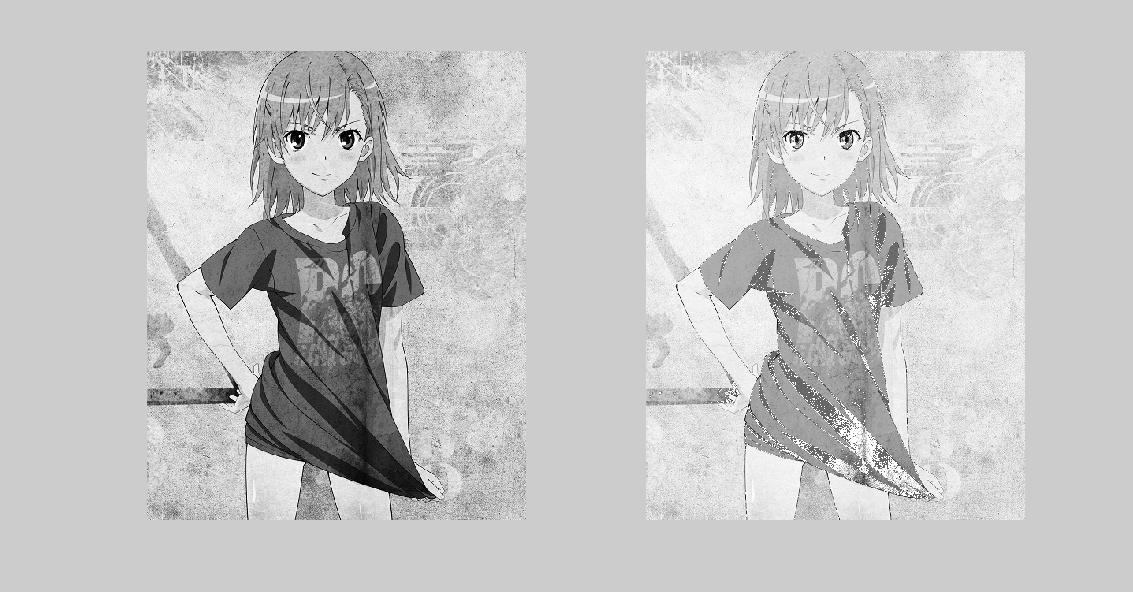3. 非线性灰度变换当输出图像的像素点灰度值和输入图像的像素点灰度值不满足线性关系时，这种灰度变换都称为非线性灰度变换，我们以对数变换的非线性变换为例，讲解非线性灰度变换。变换公式如下：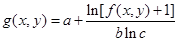close all;clear all;clc;
%基于对数变换的非线性灰度变换
R=imread('F:/pao1.jpg');
G=rgb2gray(R);  %转换成灰度图像
J=double(G);  %数据类型转换为双精度
H=(log(J+1))/10;  %进行基于常用对数的非线性灰度变换
figure,
subplot(121),imshow(G);
subplot(122),imshow(H);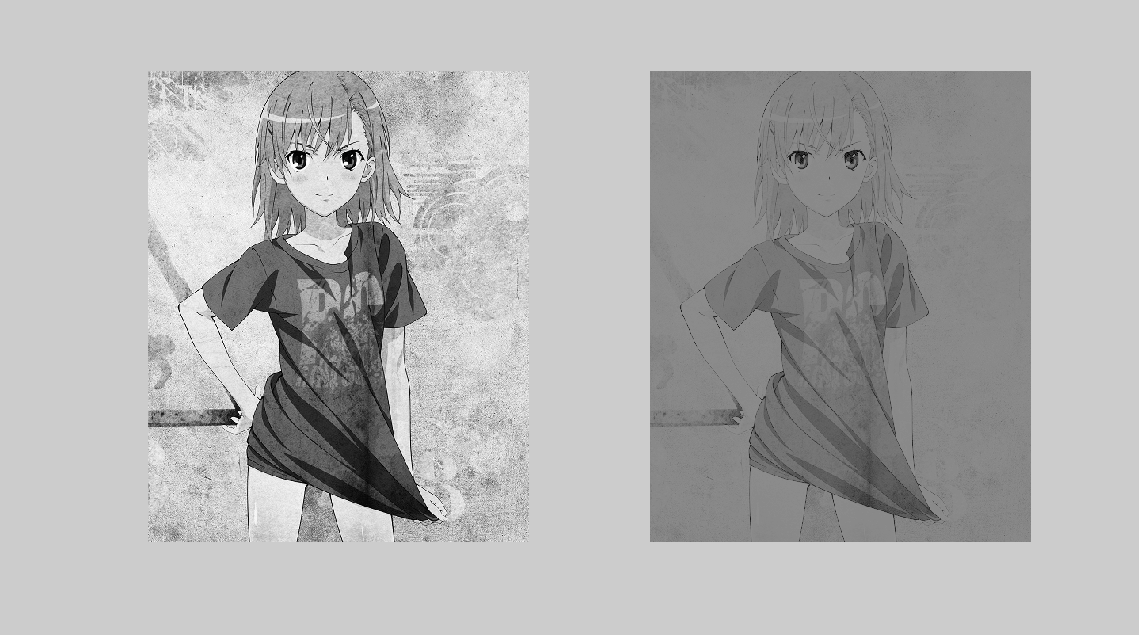总结：以上三种方法给出了三种不同类型的点运算，它们的相同之处在于都能够改变图像的显示灰度，不同之处在于采用的数学方法不同。用户在需要进行图形灰度变换时，根据实际情况选择不同的运算形式，用户可参照实例设计自己的灰度变换。------想飞上天，和太阳肩并肩------
展开全文imadjust 灰度变换
• matlab开发-图像增强平均图像质量以提高对比度版权保护。基于图像增强平均直方图均衡化的对比度增强亮度保持
• 使用matlab图像进行对比度调节，图像增强和复原的目的是为了提高图像的质量，如去除噪声，提高图像的清晰度等。图像增强不考虑图像降质的原因，突出图像中所感兴趣的部分。如强化图像高频分量，可使图像中物体轮廓...
• 图像增强中，我们遇到了使用灰度图像对比度增强，同样我们也可以在颜色空间中做同样的事情，但是这个问题会根据特定的颜色空间而有所不同，例如：RGB、CMYK 和 HSI
• 通过使用论文“Efficient Contrast Enhancement Using Adaptive Gamma Correction With Weighting Distribution”来增强图像对比度。 您可以在以下位置下载： ...
• https://blog.csdn.net/Ibelievesunshine/article/details/79947716 转载于:https://www.cnblogs.com/wojiaxiaoguo/p/9801479.html
• 题目：选择合适的基本灰度变换模型及其参数，通过该变换增强测试图像EXP2C.tif的全局对比度。...选择幂律（伽马）变换模型来增强图像对比度，幂律变换的模型为   在实验中将用X来表示伽马值γ。 步骤： 1...image
• 反锐化掩蔽滤波器（UMF）已广泛应用于图像处理前端以增强对比度。 过滤器， 易于实现，基于增强的概念图像本身的缩放和高通版本。 联动部队性能在很大程度上取决于高通的生成要添加的信号及其相关的比例因子。 然而...
• 我们无法增强彩色图像的直方图。所以我们需要将其转换为灰度，然后我们增强直方图。 通过使用直方图均衡化来增强图像对比度。 注释中提供了对使用的内置命令的简短说明。
• 数据融合matlab代码从多重曝光图像中学习深层单图像对比度增强器 抽象的 由于不良的照明条件和数字成像设备的动态范围有限，因此记录的图像通常曝光不足/曝光过度且对比度较低。 大多数以前的单图像对比度增强（SICE...
• 对比度拉伸是一种简单的图像增强技术，它试图通过“拉伸”图像所包含的强度值范围以跨越所需的值范围来提高图像对比度，例如相关图像类型允许的整个像素值范围。 它与更复杂的直方图均衡化不同，它只能对图像像素...
• 使用模糊增强的方法增加原图像对比度，以便用于后续分类和分割
• matlab对比度增强源代码，对比度增强是将图像中的亮度值范围拉伸或压缩成显示系统指定的亮度显示范围，从而提高图像全部或局部的对比度。输入图像中的每个亮度值通过一定的转换函数,对应于输出图像的一个显示值。...

# matlab增强图像对比度matlab 订阅